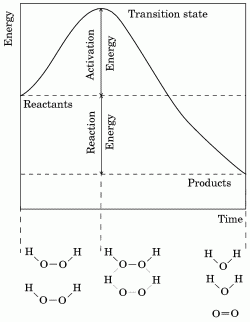# Activation energy

The activation energy in chemistry is the energy needed by a system to initiate a particular process. Activation energy is often used to denote the minimum energy needed for a specific chemical reaction to occur. For a reaction to occur between two colliding molecules, they must collide in the correct orientation, and possess a certain, minimum, amount of energy. As the molecules approach each other, their electron clouds repel each other. Overcoming this repulsion requires energy (activation energy), which is provided by the heat of the system; i.e. the translational, vibrational, and rotational energy of each molecule. If there is enough energy available, the repulsion is overcome and the molecules get close enough for attractions between the molecules to cause a rearrangement of bonds. The Arrhenius equation gives the quantitative basis of the relationship between the activation energy and the rate at which a reaction proceeds. The study of reaction rates is termed chemical kinetics.

The transition state in a reaction is the point at which the original bonds have stretched to their limit. Transition states are only in existence for extremely brief (10-15 s) periods of time. The energy required to reach the transition state is equal to the activation for that reaction. Multi-stage reactions involve a number of transition points, here the activation energy is equal to the one requiring the most energy. After this time either the molecules move apart again with original bonds reforming, or the bonds break and new products form. This is possible because both possibilities result in the release of energy (shown on the enthalpy profile diagram, Fig. 1, as both positions lie below the transition state). A substance that modifies the transition state to lower the activation energy is termed a catalyst; a biological catalyst is termed an enzyme.Fig 1. The enthalpy profile of a reaction between two hydrogen peroxide molecules to form water and oxygen. The Transition State is the point at which the original bonds have been stretched as far as they can without breaking (this is illustrated by the structural diagram below the graph.) The energy required to reach the transition state is equal to the activation energy of the reaction.

At low temperatures for a particular reaction most, but not all, molecules will not have enough energy to react. However there will nearly always be a certain number with enough energy at any temperature, because temperature is a measure of the average energy of the system - individual molecules can have more or less energy than the average. Increasing the temperature increases the proportion of molecules with more energy than the activation energy, and consequently the rate of reaction increases. Typically the activation energy is given as the energy in kilojoules needed for one mole of reactants to react.

• Art and Cultures
• Countries of the World (http://www.academickids.com/encyclopedia/index.php/Countries)
• Space and Astronomy